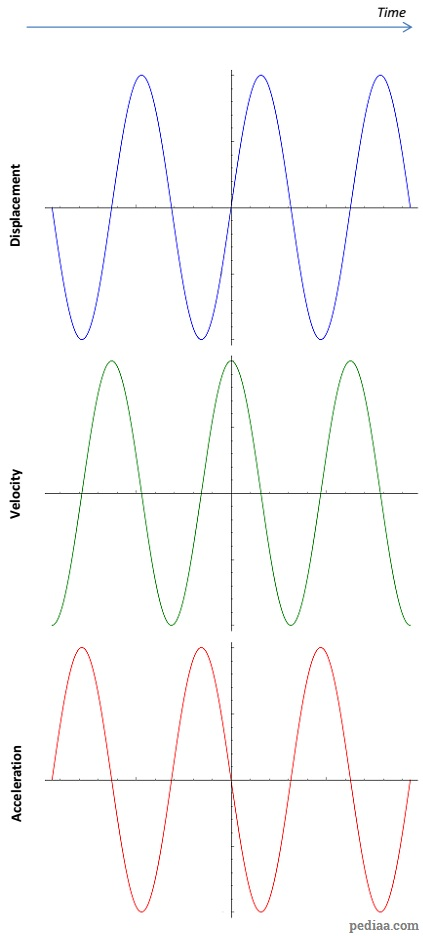# Difference Between Oscillation, Vibration and Simple Harmonic Motion

## Main Difference – Oscillation, Vibration and Simple Harmonic Motion

Oscillation, vibration and simple harmonic motion are terms that are used to describe repeated motions. The main difference between oscillation, vibration and simple harmonic motion is that oscillation refers to any repeated variation about a central value, while the term vibration refers specifically to mechanical oscillationsSimple harmonic motion refers to cases where an object oscillates about an equilibrium position under a restoring force which is directly proportional to the object’s displacement.

## What is an Oscillation

An oscillation is a periodic variation about a central value. For instance, if a child is swinging on a swing and her friend is pushing her every time the swing comes towards her, then the child’s motion can be thought of as an “oscillation”. However, the term oscillation is not a term used exclusively to describe mechanical oscillations.  For instance, neutrino oscillations refer to the fact that neutrinos regularly change their flavour: they change from electron neutrinos to muon neutrinos,  and then to tau neutrinos and back again.

## What is a Vibration

A vibration is also a type of oscillation, but the term is used to label mechanical oscillations. There are two types of mechanical vibrations: natural and forced. If you slightly displace an object that is free to move from its equilibrium position, the object will start oscillating about the equilibrium point. Here, the object will oscillate at a frequency known as the object’s natural frequency. This is an example of natural vibration. A forced vibration occurs when an external entity forces the object to move at a certain frequency. In our example of a child on a swing, if her friend pulled back the swing and let the swing move back and forth on its own, that is an example of a natural vibration. However, if the friend pushed the swing each time it came down, this is an example of a forced vibration.

## What is a Simple Harmonic Motion

Simple Harmonic Motion is a type of oscillation of an object where there is a restoring force, directly proportional to displacement, that tries to get the object back towards the equilibrium position. Consequently, the direction of the restoring force is always opposite to the direction of displacement at a given time.

It is possible to describe simple harmonic motion mathematically using trigonometric functions. The displacement of an object in simple harmonic motion can be represented mathematically using either a sine or cosine function. For instance, let us take the displacement to be:$x=A\:\mathrm{sin}\left( \omega t\right)$

The velocity of he object is then given by:$v=\frac{\mathrm{d}x}{\mathrm{d}t}=A\omega\: \mathrm{cos}\left( \omega t\right)$

Then, the acceleration becomes:$a=\frac{\mathrm{d}^2x}{\mathrm{d}t^2}=-A{\omega}^2\: \mathrm{sin}\left( \omega t\right)$

Now, we can plot these graphs to see how displacement, velocity and acceleration varies with time:How displacement, velocity and acceleration vary with time in simple harmonic motion.

The graphs reveal that the object is fastest when it is passing through the equilibrium position. From our earlier equations, we can see that this maximum velocity is$A\omega$, (where$A$ is the amplitude of displacement). Acceleration is at a maximum when the object is at its extreme position, and this maximum acceleration is given by$A{\omega}^2$.

## Difference Between Oscillation, Vibration and Simple Harmonic Motion

Oscillation is a repetitive change in a quantity about a central value.

Vibration is a mechanical oscillation.

Simple harmonic motion is a type of motion where a restoring force always acts on the oscillating object, which attempts to bring the object to the centre. The size of the restoring force is always directly proportional to the displacement.